# NAME

Chart::GGPlot - ggplot2 port in Perl

version 0.002003

# STATUS

At this moment this library is experimental and still under active development (at my after-work time). It's still quite incomplete compared to R's ggplot2 library, but the core features are working.

# SYNOPSIS

``````    use Chart::GGPlot qw(:all);
use Data::Frame::Examples qw(mtcars);

my \$plot = ggplot(
data => mtcars(),
mapping => aes( x => 'wt', y => 'mpg' )
)->geom_point();

# show in browser
\$plot->show;

# export to image file
\$plot->save('mtcars.png');

# see "examples" dir of this library's distribution for more examples.``````

# DESCRIPTION

This Chart-GGPlot library is an implementation of ggplot2 in Perl. It's designed to be possible to support multiple plotting backends. And it ships a default backend which uses Chart::Plotly.

This Chart::GGPlot module is the function interface of the Perl Chart-GGPlot library.

Example exported image files: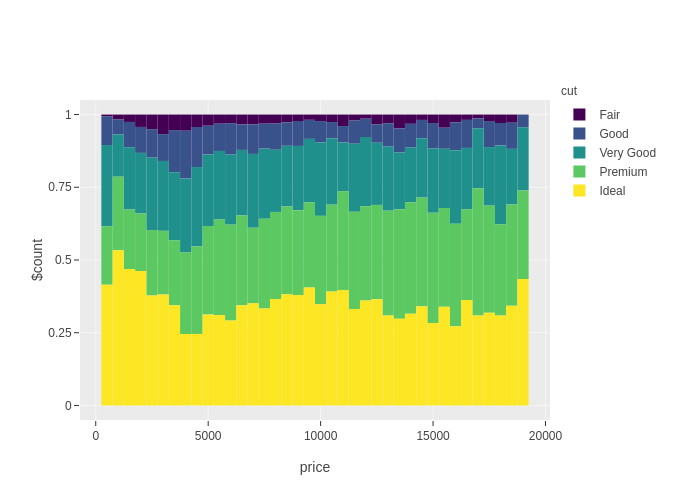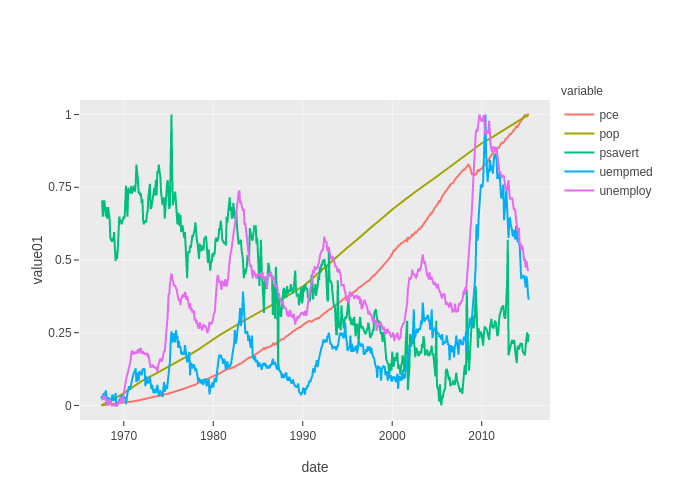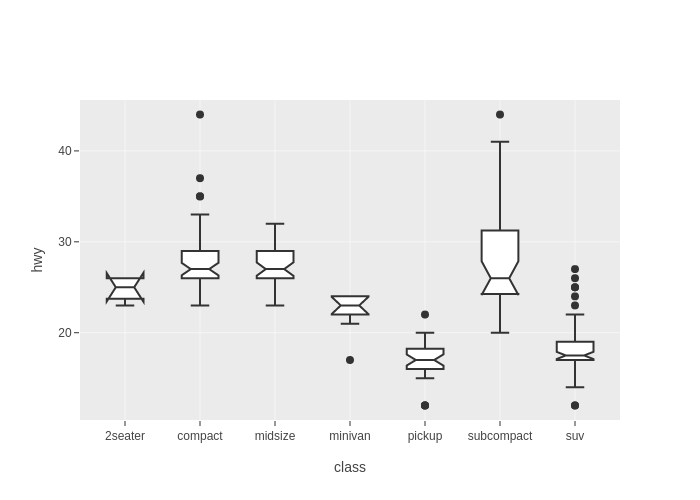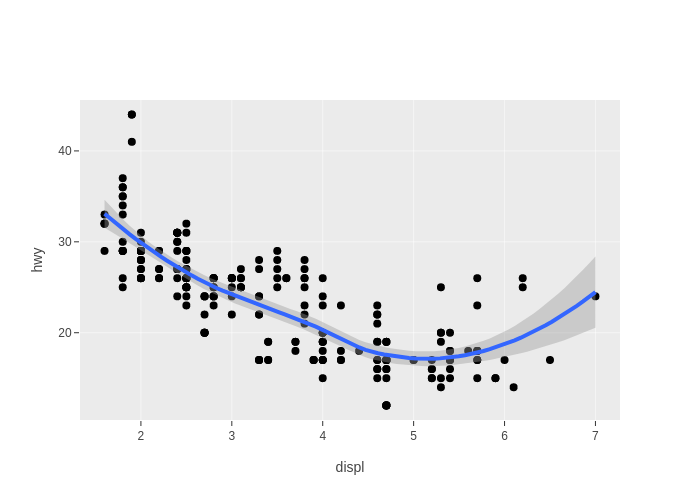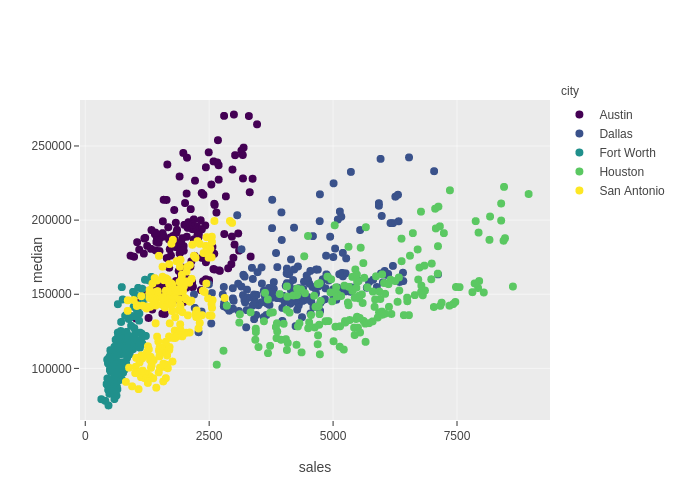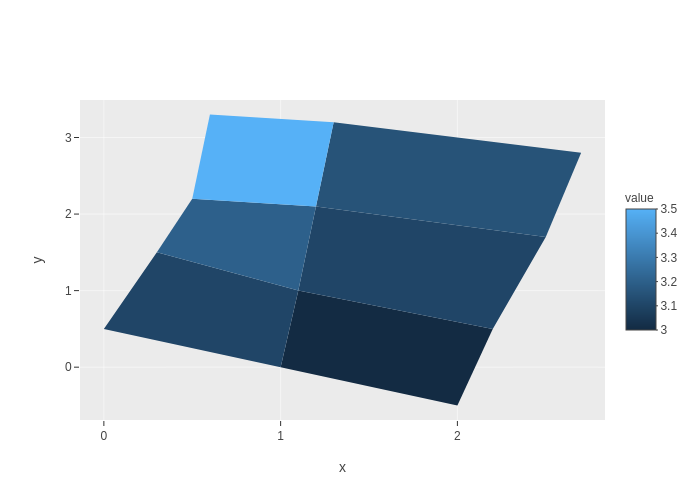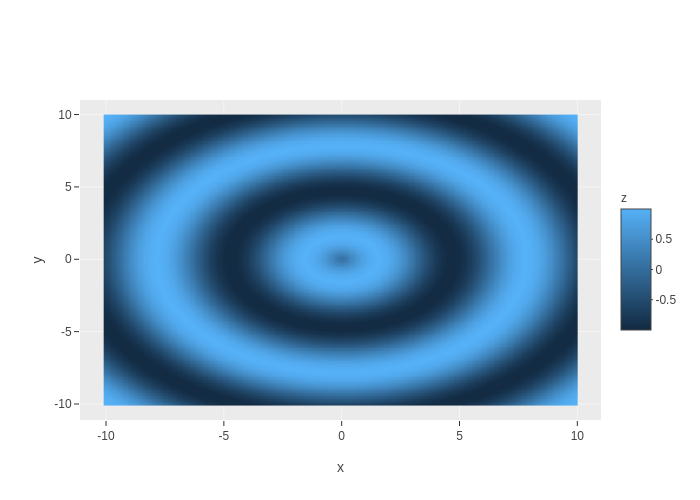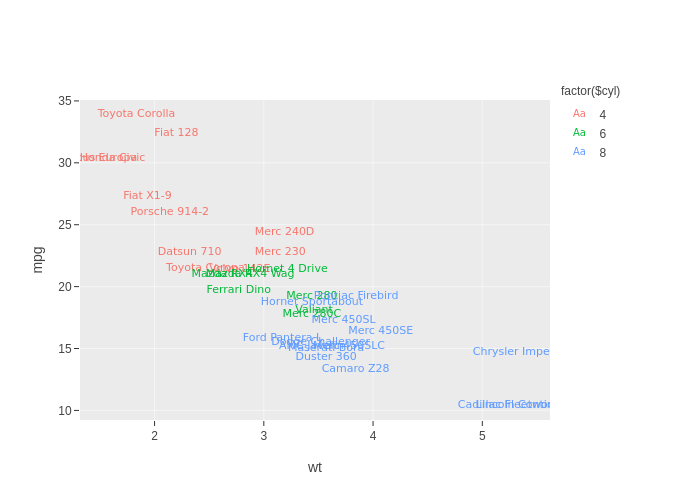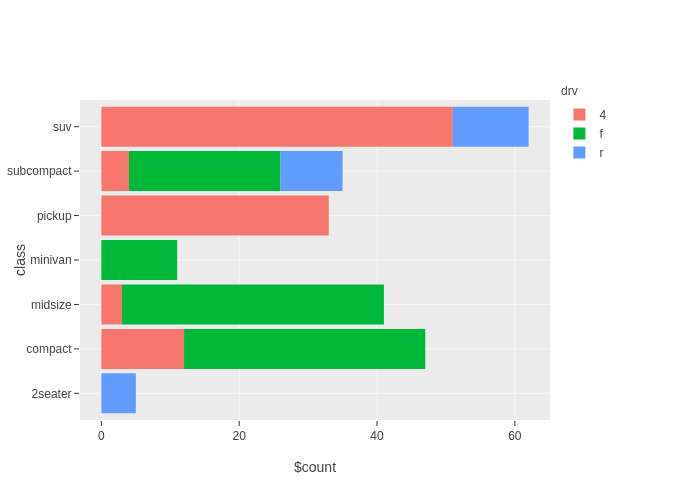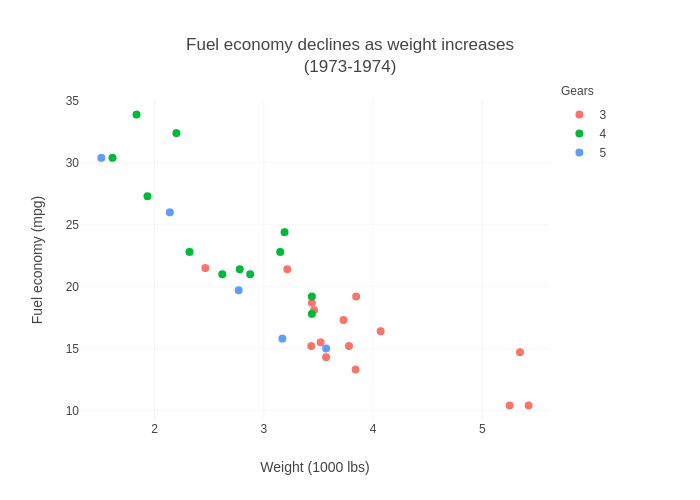See the `examples` dir in the library's distribution for more examples.

## Document Conventions

Function signatures in docs of this library follow the Function::Parameters conventions, for example,

``    myfunc(Type1 \$positional_parameter, Type2 :\$named_parameter)``

# FUNCTIONS

## ggplot

``    ggplot(:\$data, :\$mapping, %rest)``

This is same as `Chart::GGPlot::Plot->new(...)`. See Chart::GGPlot::Plot for details.

## qplot

``````    qplot((Piddle1D|ArrayRef) :\$x, (Piddle1D|ArrayRef) :\$y,
Str :\$geom='auto',
:\$xlim=undef, :\$ylim=undef,
Str :\$log='',
Maybe[Str] :\$title=undef, Str :\$xlab='x', Str :\$ylab='y',
%rest)``````

Arguments:

• \$x, \$y

Data. Supports either 1D piddles or arrayrefs. When arrayref is specified, it would be converted to either a numeric piddle or a PDL::SV piddle, guessing by its contents.

• \$geom

Geom type. `"auto"` is treated as `'point'`. It would internally call a `geom_\${geom}` function.

• \$xlim, \$ylim

Axes limits.

• \$log

Which axis use logarithmic scale? One of `''`, `'x'`, `'y'`, `'xy'`.

• \$title

Plot title. Default is `undef`, for no title.

• \$xlabel, \$ylabel

Axes labels.

# ENVIRONMENT VARIABLES

## CHART_GGPLOT_TRACE

A positive integer would enable debug messages.

ggplot2

Chart::GGPlot::Plot

Data::Frame

# AUTHOR

Stephan Loyd <sloyd@cpan.org>

# CONTRIBUTOR

Pablo Rodríguez (pablrod)